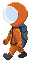Home > Books > 100 Top Tips: Microsoft Excel > Creating custom validation

# Tutorial: Creating custom validation in Excel

If your spreadsheet stores unusual data formats, such as product codes, you can use the Data Validation rules in Excel to create your own custom validation rules. Discover how to create custom rules for Data Validation in this Microsoft Excel tutorial by Sean McManus, from the book 100 Top Tips: Microsoft Excel

You can write your own validation rules for an Excel spreadsheet. Select the cell(s) you want to validate and go to the Data Validation options (see Tip 7). Select Custom in the Allow menu. In the Formula box, enter a formula that gives a result of TRUE or FALSE. Here are some examples to validate cell J8:

=COUNTIF(J8, "CPC*")=1

• Length must be 3.
=LEN(J8)=3

• Must be a number.
=ISNUMBER(J8)

• First character must be a number. The LEFT function is used to extract the character, and ISNUMBER is used to test whether it is a number. The VALUE function is required because in a data item like "UK-52", the 52 would otherwise be treated as text.
=ISNUMBER(VALUE(LEFT(J8,1)))

• Last character must not be a number. To see whether a character is not a number, we can check whether it is a number, and then wrap it in the NOT function to reverse the result.
=NOT(ISNUMBER(VALUE(RIGHT(J8,1))))

• Must be unique in column J. This shows an error if the same data has already been entered in the same column.
=COUNTIF(J:J, J8)<=1

• Must be odd. (ISEVEN can be used, too.)
=ISODD(J8).

To allow multiple options, join them with OR, like this example, which allows data of 3 or 5 characters to be valid:
=OR(LEN(J8)=3, LEN(J8)=5)

To enforce multiple validation rules, use AND, like this example, which requires a 3-character code starting with A:
=AND(LEN(J8)=3, COUNTIF(J8, "A*")=1)

## About 100 Top Tips: Microsoft Excel

This tutorial is from 100 Top Tips: Microsoft Excel. The powerful pocketbook helps you to learn more from your data, be more productive with Excel, and improve the accuracy of your spreadsheets. It's packed with shortcuts, tips, and hacks for Excel that solve everyday business problems. Find out more and download a free PDF sampler here, and order the book online here.

## CreditsTop | Search | Help | Privacy | Access Keys | Contact me